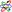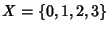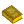## Topology

Topology is the mathematical study of properties of objects which are preserved through deformations, twistings, and stretchings. (Tearing, however, is not allowed.) A Circle is topologically equivalent to an Ellipse (into which it can be deformed by stretching) and a Sphere is equivalent to an Ellipsoid. Continuing along these lines, the Space of all positions of the minute hand on a clock is topologically equivalent to a Circle (where Space of all positions means ``the collection of all positions''). Similarly, the Space of all positions of the minute and hour hands is equivalent to a Torus. The Space of all positions of the hour, minute and second hands form a 4-D object that cannot be visualized quite as simply as the former objects since it cannot be placed in our 3-D world, although it can be visualized by other means.

There is more to topology, though. Topology began with the study of curves, surfaces, and other objects in the plane and 3-space. One of the central ideas in topology is that spatial objects like Circles and Spheres can be treated as objects in their own right, and knowledge of objects is independent of how they are ``represented'' or ``embedded'' in space. For example, the statement ``if you remove a point from a Circle, you get a line segment'' applies just as well to the Circle as to an Ellipse, and even to tangled or knotted Circles, since the statement involves only topological properties.

Topology has to do with the study of spatial objects such as curves, surfaces, the space we call our universe, the space-time of general relativity, fractals, knots, manifolds (objects with some of the same basic spatial properties as our universe), phase spaces that are encountered in physics (such as the space of hand-positions of a clock), symmetry groups like the collection of ways of rotating a top,etc.

The ``objects'' of topology are often formally defined as Topological Spaces. If two objects have the same topological properties, they are said to be Homeomorphic (although, strictly speaking, properties that are not destroyed by stretching and distorting an object are really properties preserved by Isotopy, not Homeomorphism; Isotopy has to do with distorting embedded objects, while Homeomorphism is intrinsic).

Topology is divided into Algebraic Topology (also called Combinatorial Topology), Differential Topology, and Low-Dimensional Topology.

There is also a formal definition for a topology defined in terms of set operations. A Setalong with a collectionof Subsets of it is said to be a topology if the Subsets inobey the following properties:

1. The (trivial) subsetsand the Empty Setare in.

2. Whenever setsandare in, then so is.

3. Whenever two or more sets are in, then so is their Union
(Bishop and Goldberg 1980).

A Setfor which a topologyhas been specified is called a Topological Space (Munkres 1975, p. 76). For example, the Settogether with the Subsets,,,comprises a topology, andis a Topological Space.

Topologies can be built up from Topological Bases. For the Real Numbers, the topology is the Union of Open Intervals.

See also Algebraic Topology, Differential Topology, Genus, Klein Bottle, Kuratowski Reduction Theorem, Lefshetz Trace Formula, Low-Dimensional Topology, Point-Set Topology, Zariski Topology

ReferencesTopology

Adamson, I. A General Topology Workbook. Boston, MA: Birkhäuser, 1996.

Armstrong, M. A. Basic Topology, rev. New York: Springer-Verlag, 1997.

Barr, S. Experiments in Topology. New York: Dover, 1964.

Berge, C. Topological Spaces Including a Treatment of Multi-Valued Functions, Vector Spaces and Convexity. New York: Dover, 1997.

Bishop, R. and Goldberg, S. Tensor Analysis on Manifolds. New York: Dover, 1980.

Blackett, D. W. Elementary Topology: A Combinatorial and Algebraic Approach. New York: Academic Press, 1967.

Bloch, E. A First Course in Geometric Topology and Differential Geometry. Boston, MA: Birkhäuser, 1996.

Chinn, W. G. and Steenrod, N. E. First Concepts of Topology: The Geometry of Mappings of Segments, Curves, Circles, and Disks. Washington, DC: Math. Assoc. Amer., 1966.

Eppstein, D. ``Geometric Topology.'' http://www.ics.uci.edu/~eppstein/junkyard/topo.html.

Francis, G. K. A Topological Picturebook. New York: Springer-Verlag, 1987.

Gemignani, M. C. Elementary Topology. New York: Dover, 1990.

Greever, J. Theory and Examples of Point-Set Topology. Belmont, CA: Brooks/Cole, 1967.

Hirsch, M. W. Differential Topology. New York: Springer-Verlag, 1988.

Hocking, J. G. and Young, G. S. Topology. New York: Dover, 1988.

Kahn, D. W. Topology: An Introduction to the Point-Set and Algebraic Areas. New York: Dover, 1995.

Kelley, J. L. General Topology. New York: Springer-Verlag, 1975.

Kinsey, L. C. Topology of Surfaces. New York: Springer-Verlag, 1993.

Lipschutz, S. Theory and Problems of General Topology. New York: Schaum, 1965.

Mendelson, B. Introduction to Topology. New York: Dover, 1990.

Munkres, J. R. Elementary Differential Topology. Princeton, NJ: Princeton University Press, 1963.

Munkres, J. R. Topology: A First Course. Englewood Cliffs, NJ: Prentice-Hall, 1975.

Praslov, V. V. and Sossinsky, A. B. Knots, Links, Braids and 3-Manifolds: An Introduction to the New Invariants in Low-Dimensional Topology. Providence, RI: Amer. Math. Soc., 1996.

Shakhmatv, D. and Watson, S. ``Topology Atlas.'' http://www.unipissing.ca/topology/.

Steen, L. A. and Seebach, J. A. Jr. Counterexamples in Topology. New York: Dover, 1996.

Thurston, W. P. Three-Dimensional Geometry and Topology, Vol. 1. Princeton, NJ: Princeton University Press, 1997.

van Mill, J. and Reed, G. M. (Eds.). Open Problems in Topology. New York: Elsevier, 1990.

Veblen, O. Analysis Situs, 2nd ed. New York: Amer. Math. Soc., 1946.